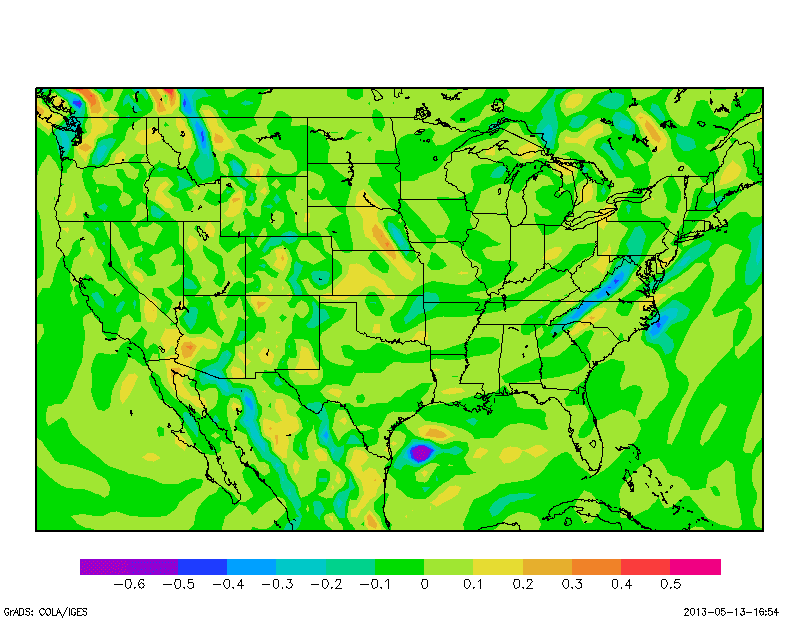## Monday, May 13, 2013

### Tips on using the finite differencing functions in GrADS (e.g., hdivg, and hcurl): Getting rid the undefined strip along the map boundary

The finite differencing functions in GrADS are pretty handy, especially when it comes to plotting variables such as divergence. The finite differencing function used in GrADS is called cdiff(), and what cdiff() does, is simply take the finite difference of a certain variable. For example, if you wanted to take the chance in u-wind in the x-direction, cdiff would be called as such:

cdiff(u,x)

It is important to note, that this is not du/dx, as it is taking the chance in u from one grid point to the next, and not the physical distance.  If you wanted du/dx, you would have to multiply the denominator by the grid-spacing.  A good example of using the cdiff function can be found here.

GrADS includes a couple of commonly needed functions that use cdiff: hdivg(), and hcurl().  These function use the finite difference method to plot horizontal divergence and vertical vorticity respectively.  A common issue that people may come across while using this function is the appearance of a "blank strip" along the boarder where the data is not plotted.  An example of this strip is below.GFS 700hPa divergence (hr-1)

It may be hard to notice here, but you can clearly see a strip of white along the boundary.  The reason this appears is simple enough, basically you are plotting a difference, and at the edge, there is only one point, so there is nothing to plot on the edge points.  The way around this is very simple.  All you need to do, is set the domain one grid point larger than you intend to plot, define your variable, then fix the domain to your plot size, and plot it.  That way, you define the finite difference at the edge of your domain before plotting.  Of course, this method relies on having the data outside of your desired domain.  Anyway, the code to do this is simple enough:

'set lat 20 52'
'set lon -127 -66'
'set mpdset hires'
'set display color white'
'clear'

'set lev 700'
'define var = hdivg(ugrdprs,vgrdprs)*3600'

'set lat 21 51'
'set lon -126 -67'

'd var'
'cbar'

As you can see the lat/lon boundaries extend out just a little bit farther when defining the variable, then are shrunk when making the actual plot.  The resulting image is:GFS 700hPA divergence (hr-1)

This image is very similar to the image above, but you now clearly see that there is no "blank" strip along the borders of the image.

The finite difference functions in GrADS can be useful for a whole number of different purposes.  Hopefully these quick tips will help you better use these functions in the future.